Examples

Chapter 8 Class 12 Application of Integrals
Serial order wise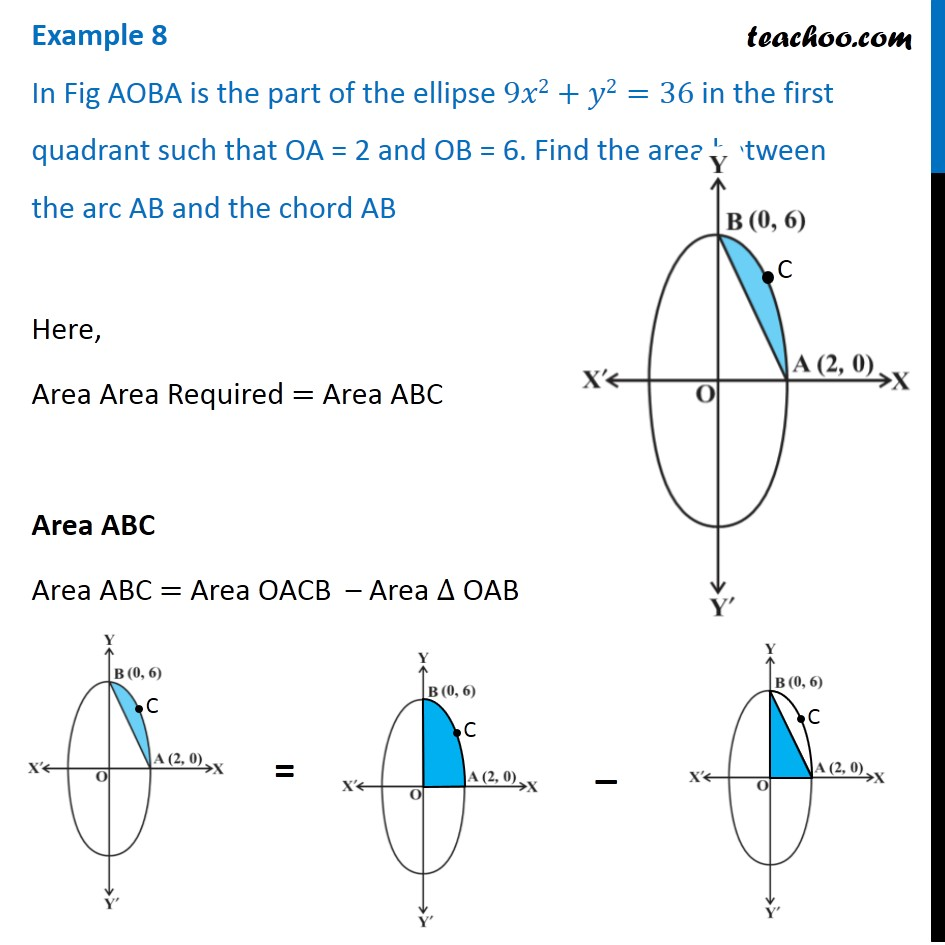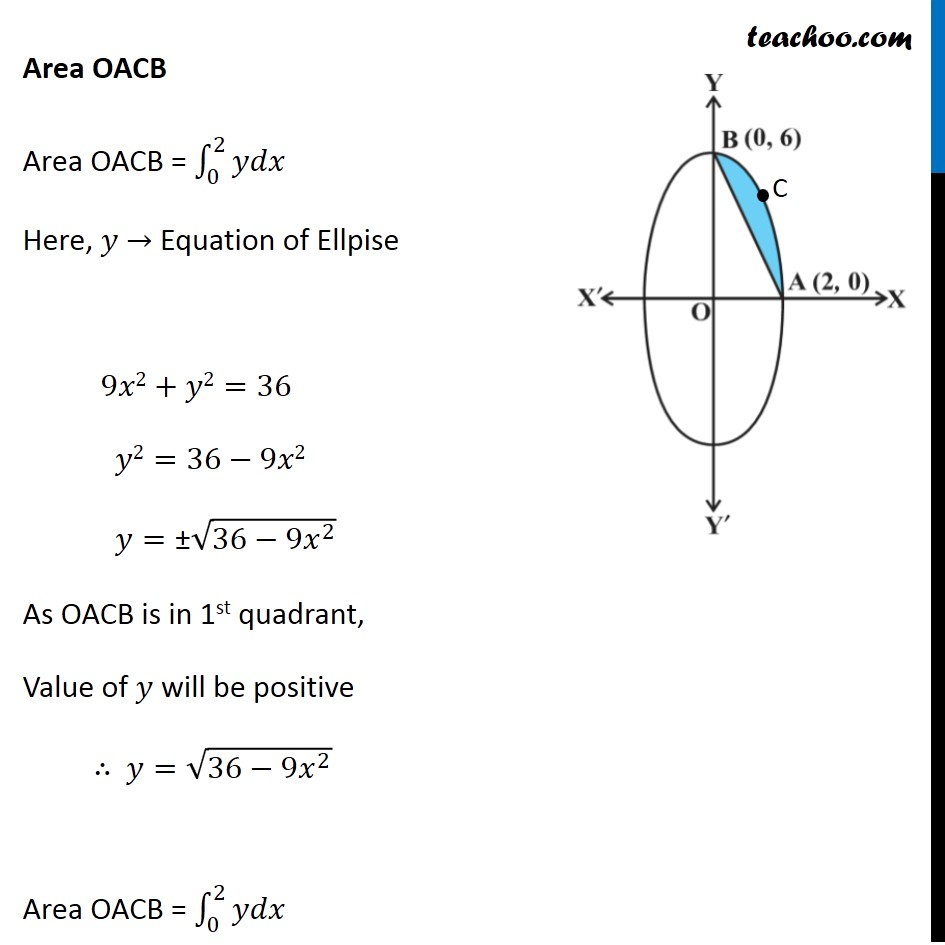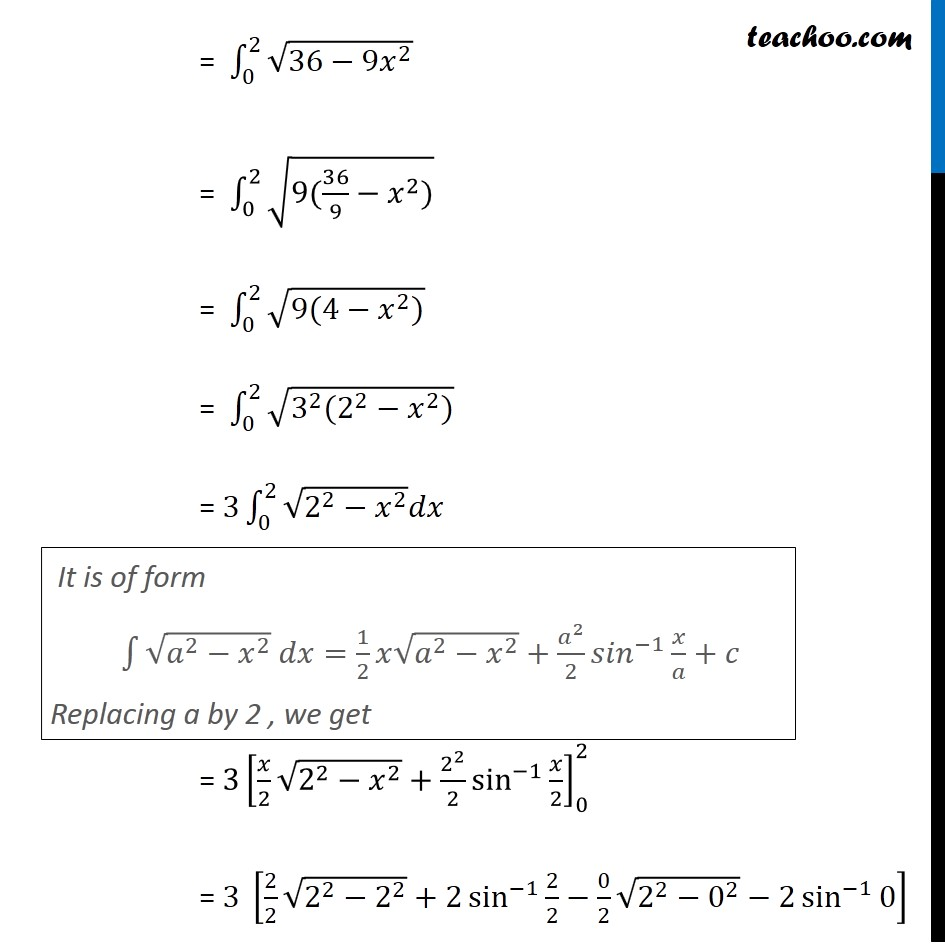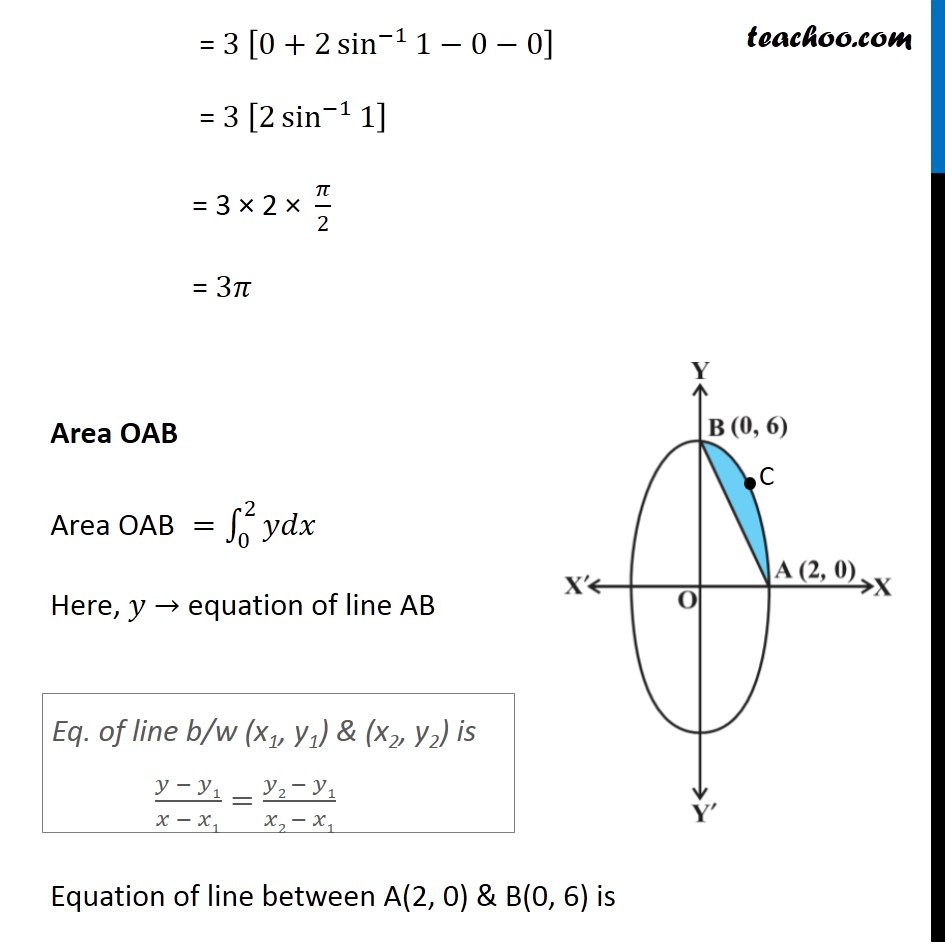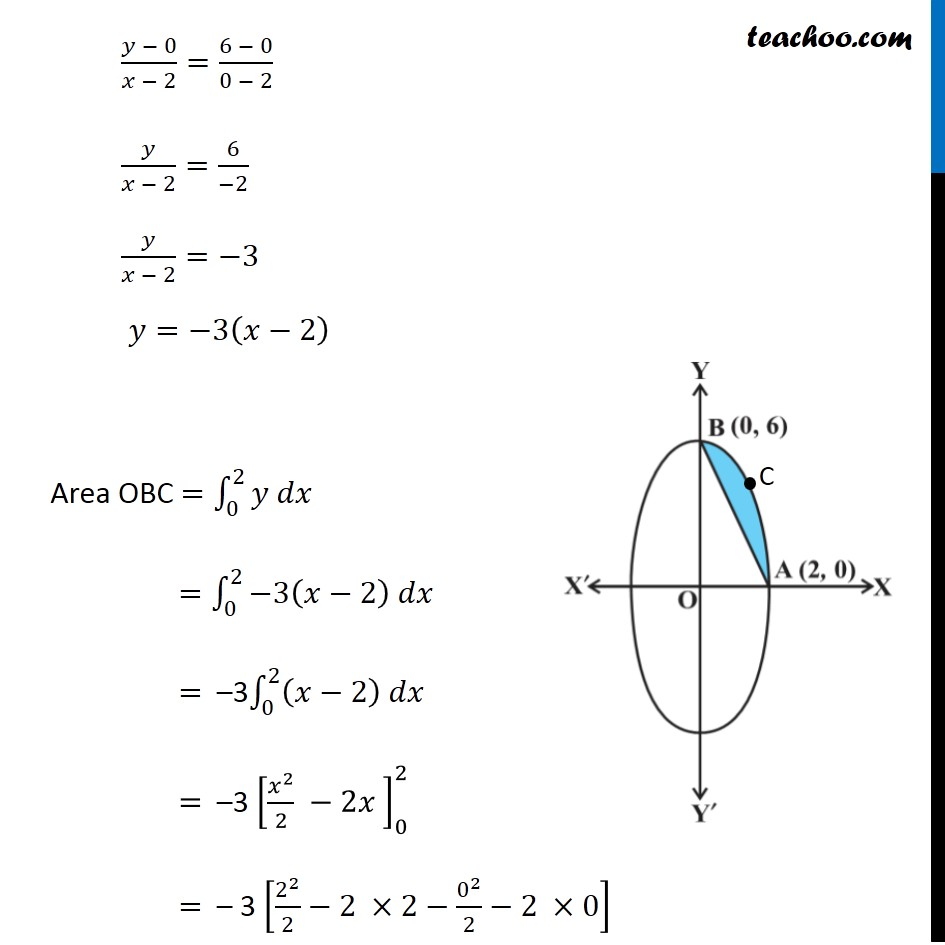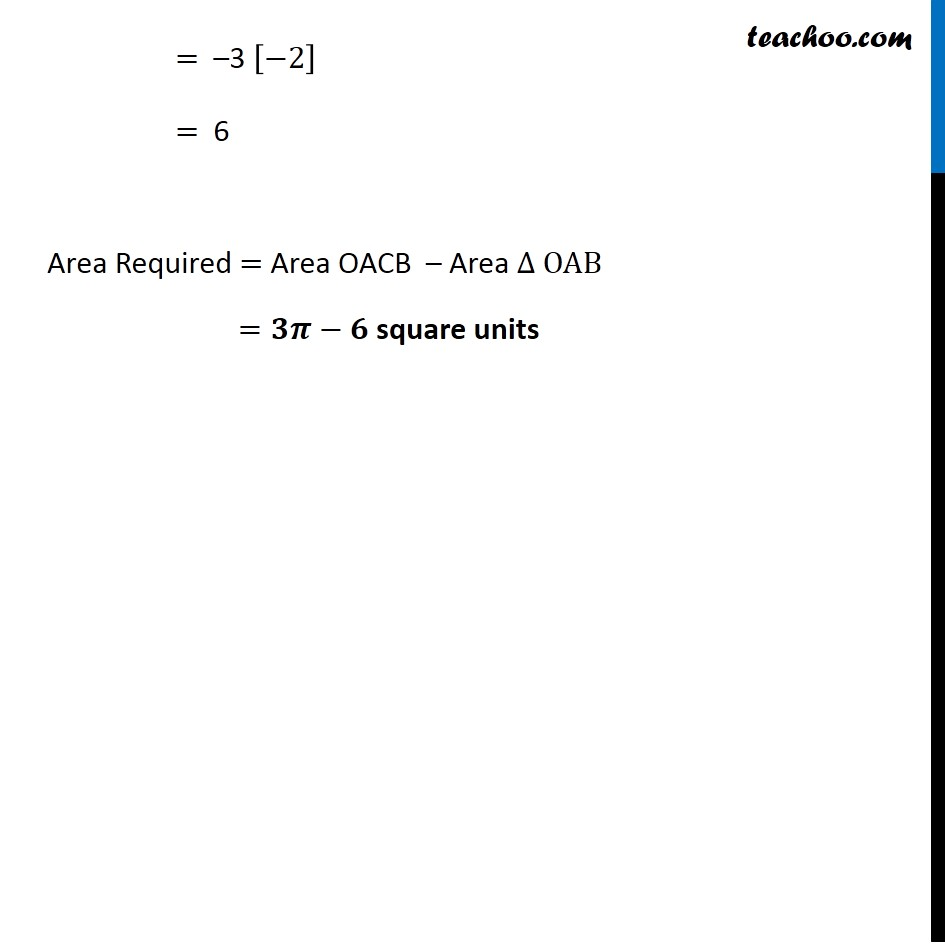Learn in your speed, with individual attention - Teachoo Maths 1-on-1 Class

### Transcript

Question 6 In Fig AOBA is the part of the ellipse 9𝑥2+𝑦2=36 in the first quadrant such that OA = 2 and OB = 6. Find the area between the arc AB and the chord AB Here, Area Area Required = Area ABC Area ABC Area ABC = Area OACB – Area ∆ OAB Area OACB Area OACB = ∫_0^2▒𝑦𝑑𝑥 Here, 𝑦 → Equation of Ellpise 9𝑥2+𝑦2=36 𝑦2=36−9𝑥2 𝑦="±" √(36−9𝑥^2 ) As OACB is in 1st quadrant, Value of 𝑦 will be positive ∴ 𝑦=√(36−9𝑥^2 ) Area OACB = ∫_0^2▒𝑦𝑑𝑥 = ∫_0^2▒√(36−9𝑥^2 ) = ∫_0^2▒√(9(36/9−𝑥^2)) = ∫_0^2▒√(9(4−𝑥^2)) = ∫_0^2▒√(3^2 (2^2−𝑥^2)) = 3∫_0^2▒〖√(2^2−𝑥^2 ) 𝑑𝑥 〗 = 3[𝑥/2 √(2^2−𝑥^2 )+2^2/2 sin^(−1)⁡〖𝑥/2〗 ]_0^2 = 3 [2/2 √(2^2−2^2 )+2 sin^(−1)⁡〖2/2−0/2〗 √(2^2−0^2 )−2 sin^(−1)⁡0 ] It is of form ∫1▒〖√(𝑎^2−𝑥^2 ) 𝑑𝑥=1/2 𝑥√(𝑎^2−𝑥^2 )〗+𝑎^2/2 〖𝑠𝑖𝑛〗^(−1)⁡〖𝑥/𝑎+𝑐〗 Replacing a by 2 , we get = 3 [0+2 sin^(−1)⁡〖1−0−0〗 ] = 3 [2 sin^(−1)⁡1 ] = 3 × 2 × 𝜋/2 = 3𝜋 Area OAB Area OAB =∫_0^2▒𝑦𝑑𝑥 Here, 𝑦 → equation of line AB Equation of line between A(2, 0) & B(0, 6) is (𝑦 − 0)/(𝑥 − 2)=(6 − 0)/(0 − 2) 𝑦/(𝑥−2)=6/(−2) Eq. of line b/w (x1, y1) & (x2, y2) is (𝑦 − 𝑦1)/(𝑥 − 𝑥1)=(𝑦2 − 𝑦1)/(𝑥2 − 𝑥1) 𝑦/(𝑥 − 2)=−3 𝑦=−3(𝑥−2) Area OBC =∫_0^2▒〖𝑦 𝑑𝑥〗 =∫_0^2▒〖−3(𝑥−2) 𝑑𝑥〗 = –3∫_0^2▒〖(𝑥−2) 𝑑𝑥〗 = –3 [𝑥^2/2 −2𝑥 ]_0^2 = – 3 [2^2/2−2 ×2−0^2/2−2 ×0] = –3 [−2] = 6 Area Required = Area OACB – Area ∆ OAB =𝟑𝝅−𝟔 square units